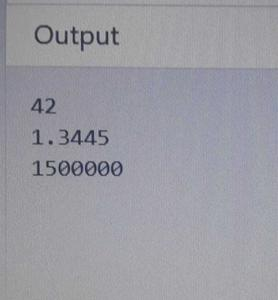Related Articles

# Difference between Decimal, Float and Double in .Net

• Difficulty Level : Hard
• Last Updated : 18 Jun, 2021

Float :
It is a floating binary point type variable. Which means it represents a number in it’s binary form. Float is a single precision 32 bits(6-9 significant figures) data type. It is used mostly in graphic libraries because of very high demand for processing power, and also in conditions where rounding errors are not very important.

Double :
It is also a floating binary point type variable with double precision and 64 bits size(15-17 significant figures). Double are probably the most generally used data type for real values, except for financial applications and places where high accuracy is desired.

Decimal :
It is a floating decimal point type variable. Which means it represents a number using decimal numbers (0-9). It uses 128 bits(28-29 significant figures) for storing and representing data. Therefore, it has more precision than float and double. They are mostly used in financial applications because of their high precision and easy to avoid rounding errors.

Example –

## C#

 `using` `System;`` ` `public` `class` `GFG {`` ` `    ``static` `public` `void` `Main()``    ``{`` ` `        ``double` `d = 0.42e2;    ``//double data type``        ``Console.WriteLine(d); ``// output 42`` ` `        ``float` `f = 134.45E-2f;  ``//float data type``        ``Console.WriteLine(f); ``// output: 1.3445`` ` `        ``decimal` `m = 1.5E6m;   ``//decimal data type``        ``Console.WriteLine(m); ``// output: 1500000``    ``}``}`

Output –Example of double, float and decimal

Comparison between Float, Double and Decimal on the Basis of :

• No. of Bits used –
1. Float uses 32 bits to represent data.
2. Double uses 64 bits to represent data.
3. Decimal uses 128 bits to represent data.
• Range of values –
1. The float value ranges from approximately ±1.5e-45 to ±3.4e38.
2. The double value ranges from approximately ±5.0e-324 to ±1.7e308.
3. The Decimal value ranges from approximately ±1.0e-28 to ±7.9e28.
• Precision –
1. Float represent data with single precision.
2. Double represent data with double precision.
3. Decimal has higher precision than float and Double.
• Accuracy –
1. Float is less accurate than Double and Decimal.
2. Double is more accurate than Float but less accurate than Decimal.
3. Decimal is more accurate than Float and Double.

For documentation related to Float , double and Decimal, kindly visit here.

Attention reader! Don’t stop learning now.  Practice GATE exam well before the actual exam with the subject-wise and overall quizzes available in GATE Test Series Course.

Learn all GATE CS concepts with Free Live Classes on our youtube channel.

My Personal Notes arrow_drop_up